Question

3)      Consider [Co(H2O)6]2+   and [Cr(C2O4)3]3-   complex ions

a)       Calculate the splitting energies of these complex ions.

b)      Compare your results with pairing energies of these metal ions and decide whether the ligands are weak field or strong field ligands.

c)       Calculate the crystal field stabilization energy for each compound.

 Complex λ1 λ2 λ3 Pairing   Energy (Kj/mol) [Co(H2O)6]2+ 510 460 - 250. 0 [Cr(C2O4)3]3- 565 415 266 244.3

We need at least 10 more requests to produce the answer.

0 / 10 have requested this problem solution

The more requests, the faster the answer.

All students who have requested the answer will be notified once they are available.

#### Earn Coins

Coins can be redeemed for fabulous gifts.

Similar Homework Help Questions
• ### Calculate the crystal field stabilization energy valiue for the complex Cr(NH)J*. The crystal field splitting energy...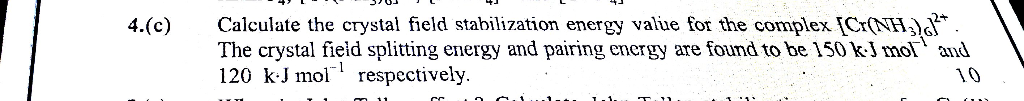Calculate the crystal field stabilization energy valiue for the complex Cr(NH)J*. The crystal field splitting energy and pairing energy are found to be 150 kJ mol and 120 kJ mol respectively. 4.(c) 10 cc. Calculate the crystal field stabilization energy valiue for the complex Cr(NH)J*. The crystal field splitting energy and pairing energy are found to be 150 kJ mol and 120 kJ mol respectively. 4.(c) 10 cc.

• ### Date: 1117 mework 10 - Ch. 21-23 A) CN B)NO c)r D) CO Name: E ) en 24. Place the ligands, CN-, Br., H2O, and OH-in o...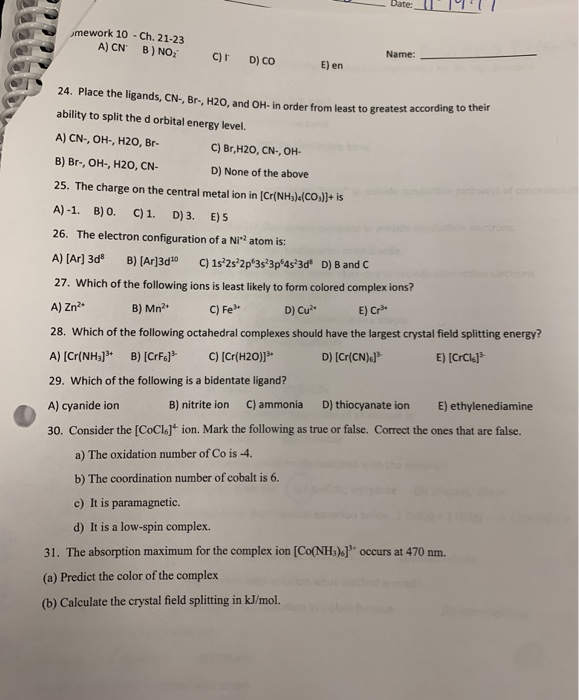Date: 1117 mework 10 - Ch. 21-23 A) CN B)NO c)r D) CO Name: E ) en 24. Place the ligands, CN-, Br., H2O, and OH-in order from least to greatest according ability to split the d orbital energy level. A) CN-, OH, H2O, Br- C) Br,H20, CN-, OH- B) Br., OH, H2O, CN- D) None of the above 25. The charge on the central metal ion in (Cr(NH3)(CO3)2+ is A)-1. B) 0. C) 1. D) 3. E) 5 26....

• ### a. Predict the structure of [Cr(OH2)6]2+ - I wrote that the complex will undergo Jahn-Teller distortion,...

a. Predict the structure of [Cr(OH2)6]2+ - I wrote that the complex will undergo Jahn-Teller distortion, making the octahedral complex become tetragonal (don't know if that's correct) b. Account for the variation in the lattice enthalpy of Mn (2780 kJ/mol), Fe (2926 kJ/mol), Co (2976 kJ/mol), Ni (3060 kJ/mol), and Zn (2985 kJ/mol) flouride given that the metal center in all of the flourides is surrounded by an octahedral array of F-ions. c. Explain the effect on the d-orbital energies...

• ### Q1. The following species a)-c) all contain complex ions of transition metals, which you can assume...

Q1. The following species a)-c) all contain complex ions of transition metals, which you can assume take up an octahedral geometry. a) [RuCl3(H2O)3] b) [Ir(bpy)3]Cl3 ; bipy = 2,2'-bipyridine c) K2[Mo(NCS)6] For each species: i) draw all the possible isomers of the complex ions; ii) calculate the charge of the metal, and the dn configuration; iii) draw a diagram showing d orbital occupancy and, where high and low spin cases are possible, predict which will be favoured considering the spectrochemical...

• ### .. Fe is identified by the rapid formation of the intensely colored pentaaquathio-cyanatoiron (III) complex ion,...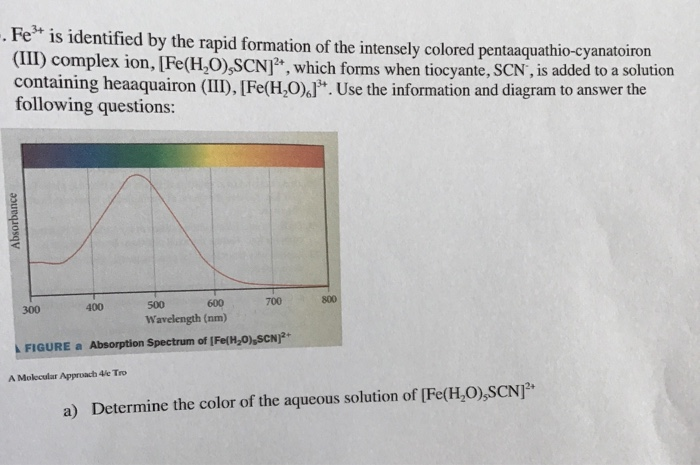.. Fe is identified by the rapid formation of the intensely colored pentaaquathio-cyanatoiron (III) complex ion, [Fe(H,O),SCN , which forms when tiocyante, SCN, is added to a solution containing heaaquairon (III), [Fe(H,0).J. Use the information and diagram to answer the following questions: Absorbance 300 400 500 600 700 Wavelength (nm) FIGURE a Absorption Spectrum of [Fe(H20),SCN)2+ A Molecular Approach 4le Tro a) Determine the color of the aqueous solution of [Fe(HO),SCN b) Calculate the crystal field splitting energy of [Fe(H,0),SCN]...

• ### can anyone please confirm my answers for 5&6? And can you explain how to fill in...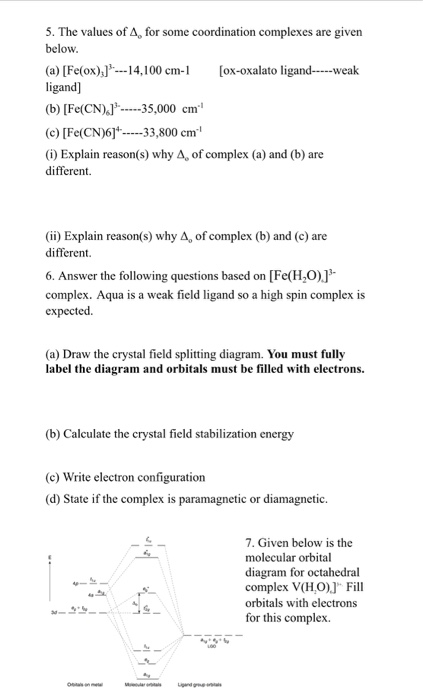can anyone please confirm my answers for 5&6? And can you explain how to fill in the electrons for 7? Please and Thank you. 5. The values of A, for some coordination complexes are given below. (a) [Fe(ox)) --- 14,100 cm-1 (ox-oxalato ligand-----weak ligand] (b) [Fe(CN).-----35,000 cm ©) [Fe(CN)6]* -----33,800 cm (1) Explain reason(s) why A. of complex (a) and (b) are different (ii) Explain reason(s) why 4, of complex (b) and (c) are different 6. Answer the following questions...

• ### 1. What is the crystal field stabilisation energy (CFSE) of a d5 metal ion in an...

1. What is the crystal field stabilisation energy (CFSE) of a d5 metal ion in an octahedral ligand field surrounded by weak field ligands? +5Dq -20Dq 0Dq -5Dq -20Dq + P 2. The relative ordering of the d orbital energy levels for a complex such as [Cu(H2O)6]2+ which exhibits tetragonal elongation due to the Jahn-Teller Effect is: Group of answer choices xz = yz = xy < x2-y2 = z2 xy < xz = yz < x2-y2 < z2 xz...

• ### do all Question 3 1 pts The complex [Os(bpy)2C12]* can exist as how many geometric and...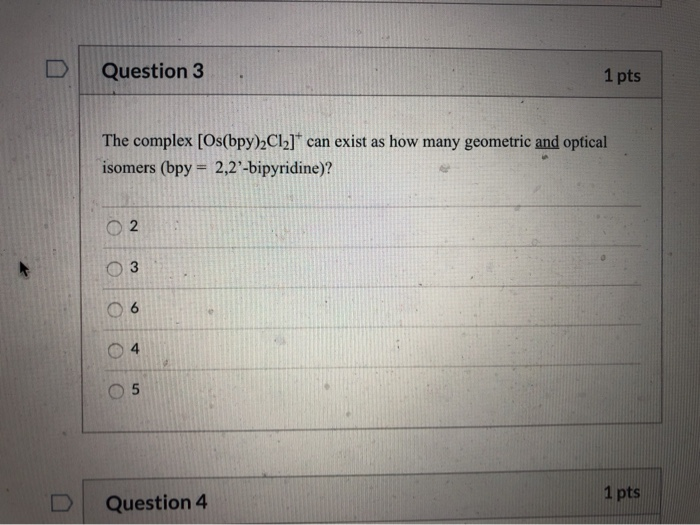do all Question 3 1 pts The complex [Os(bpy)2C12]* can exist as how many geometric and optical isomers (bpy = 2,2-bipyridine)? 03 O 5 Question 4 1 pts Question 5 1 pts The lowest energy absorption band (which corresponds to 10Dq) for [V(OH2)6]2+ occurs at 12,300 cm-'. The crystal field stabilisation energy (CFSE) for [V(OH2)]2+ is approximately 4,920 cm 7,380 cm 9,840 cm 12,300 cm 14,700 cm 1 pts Question 6 Question 6 1 pts Arrange the ligands in the...

• ### In the complex ion [ML6]n+, Mn+ has five d electrons and L is a strong field...

In the complex ion [ML6]n+, Mn+ has five d electrons and L is a strong field ligand. According to crystal field theory, the magnetic properties of the complex ion correspond to how many unpaired electrons? A. 0 B. 1 C. 2 D. 3 E. 5 Which one of these complex ions would absorb light with the shortest wavelength? A. [Co(H2O)6]2+ B. [Co(NH3)6]2+ C. [CoF6]4– D. [Co(CN)6]4– E. [Co(en)6]2+ The ion [Co(NH3)6]2+ is octahedral and high spin. This complex is (4pts)...

• ### i can't solve this question of coordination chemistry, could someone hlep me please Exercise 1: 1. What kind(s) o...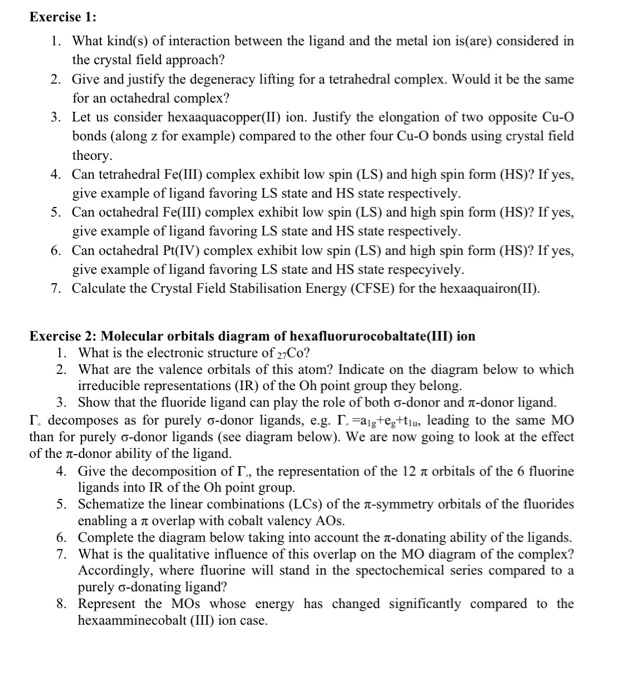i can't solve this question of coordination chemistry, could someone hlep me please Exercise 1: 1. What kind(s) of interaction between the ligand and the metal ion is(are) considered in the crystal field approach? 2. Give and justify the degeneracy lifting for a tetrahedral complex. Would it be the same for an octahedral complex? 3. Let us consider hexaaquacopper(II) ion. Justify the elongation of two opposite Cu-O bonds (along z for example) compared to the other four Cu-O bonds using...